# Test: Areas Of Parallelograms And Triangles- 2

## 25 Questions MCQ Test Mathematics (Maths) Class 9 | Test: Areas Of Parallelograms And Triangles- 2

Description
This mock test of Test: Areas Of Parallelograms And Triangles- 2 for Class 9 helps you for every Class 9 entrance exam. This contains 25 Multiple Choice Questions for Class 9 Test: Areas Of Parallelograms And Triangles- 2 (mcq) to study with solutions a complete question bank. The solved questions answers in this Test: Areas Of Parallelograms And Triangles- 2 quiz give you a good mix of easy questions and tough questions. Class 9 students definitely take this Test: Areas Of Parallelograms And Triangles- 2 exercise for a better result in the exam. You can find other Test: Areas Of Parallelograms And Triangles- 2 extra questions, long questions & short questions for Class 9 on EduRev as well by searching above.
QUESTION: 1

### D,E,F are mid points of the sides BC, CA & AB respectively of ΔABC, then area of BDEF is equal to

Solution: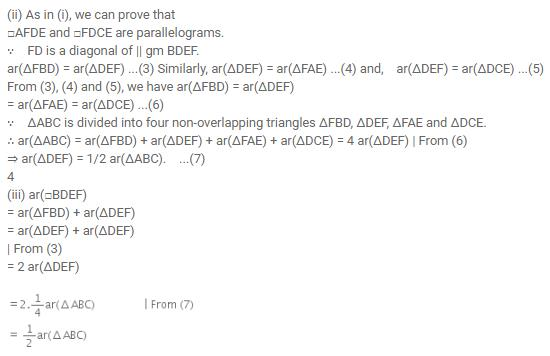QUESTION: 2

Solution:
QUESTION: 3

### The median of a triangle divides it into two

Solution:
QUESTION: 4

The area of the figure formed by joining the mid-points of the adjacent sides of a rhombus with diagonals 16 cm and 12 cm is

Solution: The fig. formed by joining mid points is parellelogram and area of this parellelogram = 1/2 of the area of rhombs Area of rhombus= 1 /2x12x16 =96;;;;;; or Area of parellelogram= 1/2x96 = 48cm^2
QUESTION: 5

The figure obtained by joining the mid-points of the adjacent sides of a rectangle of sides 8 cm and 6 cm is

Solution:
QUESTION: 6

ABCD is quadrilateral whose diagonal AC divides it into two parts, equal in area, then ABCD

Solution:

Here, ABCD need not be any of rectangle, rhombus and parallelogram because if ABCD is a square, then its diagonal AC also divides it into two parts which are equal in area.

QUESTION: 7

Two parallelograms are on equal bases and between the same parallels. The ratio of their areas is

Solution:
QUESTION: 8

If a triangle and a parallelogram are on the same base and between the same parallels, then the ratio of the area of the triangle to the area of the parallelogram is

Solution:
QUESTION: 9

ABCD is a parallelogram. If AB = 12 cm, AE 7.5 cm, CF = 15 cm, then AD is equal to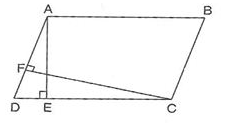Solution:
QUESTION: 10

A, B, C, D are mid-points of sides of parallelogram PQRS. If ar(PQRS)=36cm2, then ar (ABCD) is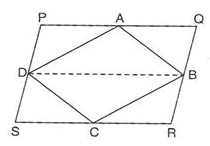Solution:
QUESTION: 11

In the given figure if ar(ΔABCD) = 29cm2 and AB = 5.8 cm, then the height of ΔABEF is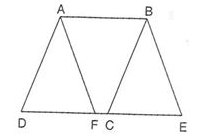Solution:
QUESTION: 12

AP||BQ||CR. If ar(ΔAQC)=17cm2, then ar(ΔPBR) is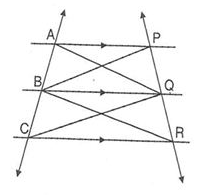Solution:
QUESTION: 13

ABCD is a square. P and Q are mid-point of AB and DC respectively. If AB = 8 cm, then ar(ΔBPD) is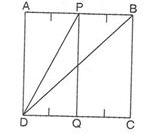Solution:
QUESTION: 14

PQRS is a parallelogram. If X and Y are mid-points of PQ and SR and diagonal SQ is joined, then ar(ΔXQRY): ar(ΔQSR) is equal to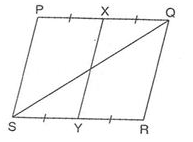Solution:
QUESTION: 15

ABCD is a trapezium with parallel sides AB = a cm and DC = b cm. E and F are the mid-points of the non-parallel sides. The ratio of ar (ABFE) to ar (EFCD) is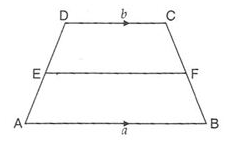Solution:
QUESTION: 16

In quadrilateral PQRS, M is the mid-point of PR. If ar (SMQR) is 18cm2, then ar (PQMS) is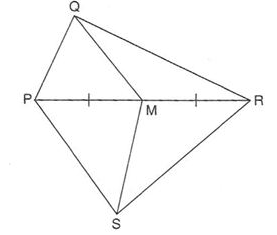Solution:
QUESTION: 17

In the given figure ABCD is a parallelogram and its area is 64cm2. If P is any point in the interior of ΔABCD, then ar(ΔAPD)+ar(ΔPBC) is equal to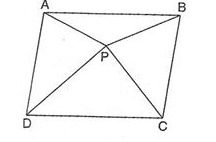Solution:
QUESTION: 18

D and E are mid-points of BC and AD respectively. If ar(ΔABC) = 10cm2, then ar(ΔEBC) is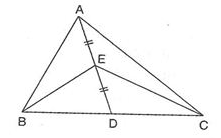Solution:
QUESTION: 19

If E and F are mid-points of sides AB and CD respectively and ar(ΔABCD) = 36cm2,then ar(APD): ar(DEF)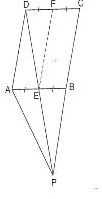Solution:
QUESTION: 20

In the given figure, if BC║AE,CD║BE, and ar(ΔBED) = 6cm2, then ar(ΔABC) is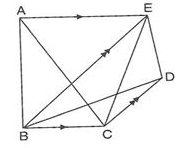Solution:
QUESTION: 21

PQRS is a parallelogram. A and B are any points on PQ and RQ respectively. If ar(ΔSBR) = 16cmand ar(ΔPBQ) = 8cm2, then the area of ΔRAS is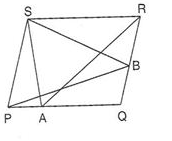Solution:
QUESTION: 22

ABCD is a parallelogram. P is any point on CD. If ar(ΔDPA) = 15cm2 and ar(ΔAPC)=20cm2, thenar(ΔAPB)is

Solution:
QUESTION: 23

M and N are the mid-points of sides DC and AB respectively, of a rectangle ABCD. If ar(rectangle ABCD) = 48cm2, then ar(ΔEMC) is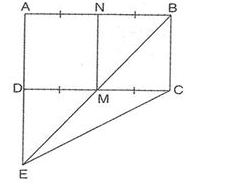Solution:
QUESTION: 24

ABCD is a trapezium in which AB║DC.AB║DC. A line through A parallel to BC meets diagonal BD at P. If ar(ΔBPC) = 5cm2, then ar(ΔABD) is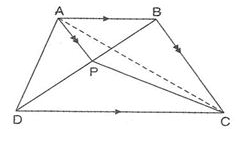Solution:
QUESTION: 25

PQRS and ADEQ are rectangles. RE║AP. If ar(ACPQ) = 25cm2 and ar(ABEP) = 10cm2,then ar (PQRS) is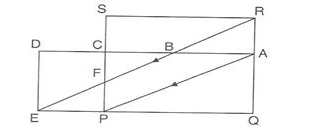Solution: